# Multi-Class Fault Detection Using Simulated Data

This example shows how to use a Simulink model to generate fault and healthy data. The data is used to develop a multi-class classifier to detect different combinations of faults. The example uses a triplex reciprocating pump model and includes leak, blocking, and bearing faults.

### Setup the Model

This example uses many supporting files that are stored in a zip file. Unzip the file to get access to the supporting files, load the model parameters, and create the reciprocating pump library.

```if ~exist('+mech_hydro_forcesPS','dir') unzip('pdmRecipPump_supportingfiles.zip') end % Load Parameters pdmRecipPump_Parameters %Pump CAT_Pump_1051_DataFile_imported %CAD % Create Simscape library if needed if exist('mech_hydro_forcesPS_Lib','file')~=4 ssc_build mech_hydro_forcesPS end```

### Reciprocating Pump Model

The reciprocating pump consists of an electric motor, the pump housing, pump crank and pump plungers.

```mdl = 'pdmRecipPump'; open_system(mdl)```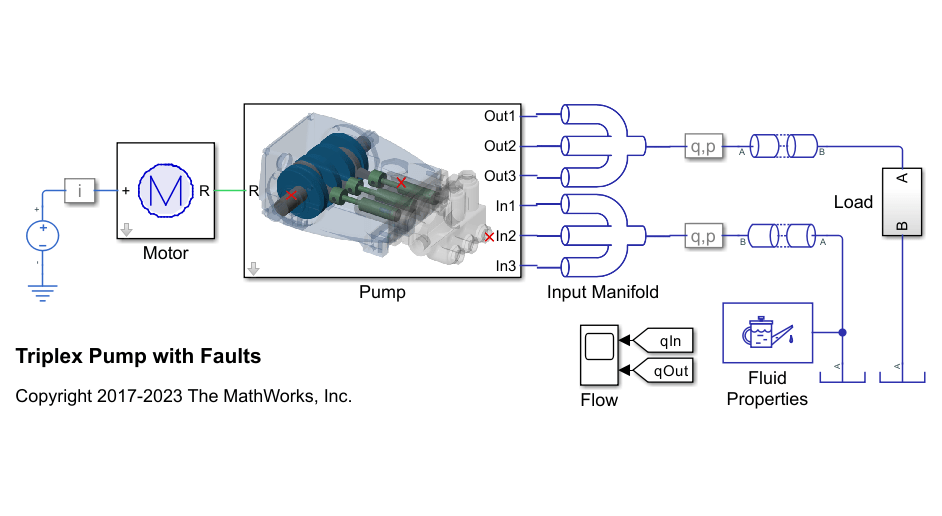`open_system([mdl,'/Pump'])`The pump model is configured to model three types of faults; cylinder leaks, blocked inlet, and increased bearing friction. These faults are parameterized as workspace variables and configured through the pump block dialog.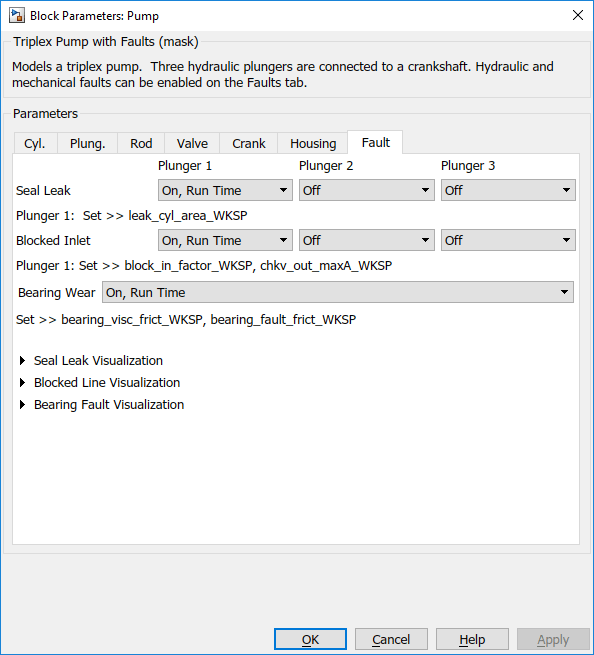### Simulating Fault and Healthy Data

For each of the three fault types create an array of values that represent the fault severity, ranging from no fault to a significant fault.

```% Define fault parameter variations numParValues = 10; leak_area_set_factor = linspace(0.00,0.036,numParValues); leak_area_set = leak_area_set_factor*TRP_Par.Check_Valve.In.Max_Area; leak_area_set = max(leak_area_set,1e-9); % Leakage area cannot be 0 blockinfactor_set = linspace(0.8,0.53,numParValues); bearingfactor_set = linspace(0,6e-4,numParValues);```

The pump model is configured to include noise, thus running the model with the same fault parameter values will result in different simulation outputs. This is useful for developing a classifier as it means there can be multiple simulation results for the same fault condition and severity. To configure simulations for such results, create vectors of fault parameter values where the values represent no faults, a single fault, combinations of two faults, and combinations of three faults. For each group (no fault, single fault, etc.) create 125 combinations of fault values from the fault parameter values defined above. This gives a total of 1000 combinations of fault parameter values.

```nPerGroup = 125; % Number of elements in each fault group % No fault simulations leakArea = repmat(leak_area_set(1),nPerGroup,1); blockingFactor = repmat(blockinfactor_set(1),nPerGroup,1); bearingFactor = repmat(bearingfactor_set(1),nPerGroup,1); % Single fault simulations idx = ceil(10*rand(nPerGroup,1)); leakArea = [leakArea; leak_area_set(idx)']; blockingFactor = [blockingFactor;repmat(blockinfactor_set(1),nPerGroup,1)]; bearingFactor = [bearingFactor;repmat(bearingfactor_set(1),nPerGroup,1)]; idx = ceil(10*rand(nPerGroup,1)); leakArea = [leakArea; repmat(leak_area_set(1),nPerGroup,1)]; blockingFactor = [blockingFactor;blockinfactor_set(idx)']; bearingFactor = [bearingFactor;repmat(bearingfactor_set(1),nPerGroup,1)]; idx = ceil(10*rand(nPerGroup,1)); leakArea = [leakArea; repmat(leak_area_set(1),nPerGroup,1)]; blockingFactor = [blockingFactor;repmat(blockinfactor_set(1),nPerGroup,1)]; bearingFactor = [bearingFactor;bearingfactor_set(idx)']; % Double fault simulations idxA = ceil(10*rand(nPerGroup,1)); idxB = ceil(10*rand(nPerGroup,1)); leakArea = [leakArea; leak_area_set(idxA)']; blockingFactor = [blockingFactor;blockinfactor_set(idxB)']; bearingFactor = [bearingFactor;repmat(bearingfactor_set(1),nPerGroup,1)]; idxA = ceil(10*rand(nPerGroup,1)); idxB = ceil(10*rand(nPerGroup,1)); leakArea = [leakArea; leak_area_set(idxA)']; blockingFactor = [blockingFactor;repmat(blockinfactor_set(1),nPerGroup,1)]; bearingFactor = [bearingFactor;bearingfactor_set(idxB)']; idxA = ceil(10*rand(nPerGroup,1)); idxB = ceil(10*rand(nPerGroup,1)); leakArea = [leakArea; repmat(leak_area_set(1),nPerGroup,1)]; blockingFactor = [blockingFactor;blockinfactor_set(idxA)']; bearingFactor = [bearingFactor;bearingfactor_set(idxB)']; % Triple fault simulations idxA = ceil(10*rand(nPerGroup,1)); idxB = ceil(10*rand(nPerGroup,1)); idxC = ceil(10*rand(nPerGroup,1)); leakArea = [leakArea; leak_area_set(idxA)']; blockingFactor = [blockingFactor;blockinfactor_set(idxB)']; bearingFactor = [bearingFactor;bearingfactor_set(idxC)'];```

Use the fault parameter combinations to create `Simulink.SimulationInput` objects. For each simulation input ensure that the random seed is set differently to generate different results.

```for ct = numel(leakArea):-1:1 simInput(ct) = Simulink.SimulationInput(mdl); simInput(ct) = setVariable(simInput(ct),'leak_cyl_area_WKSP',leakArea(ct)); simInput(ct) = setVariable(simInput(ct),'block_in_factor_WKSP',blockingFactor(ct)); simInput(ct) = setVariable(simInput(ct),'bearing_fault_frict_WKSP',bearingFactor(ct)); simInput(ct) = setVariable(simInput(ct),'noise_seed_offset_WKSP',ct-1); end```

Use the `generateSimulationEnsemble` function to run the simulations defined by the `Simulink.SimulationInput` objects defined above and store the results in a local sub-folder. Then create a `simulationEnsembleDatastore` from the stored results.

Note that running these 1000 simulations in parallel takes around an hour on a standard desktop and generates around 620MB of data. An option to only run the first 10 simulations is provided for convenience.

```% Run the simulation and create an ensemble to manage the simulation results runAll = true; if runAll [ok,e] = generateSimulationEnsemble(simInput,fullfile('.','Data'),'UseParallel',true); else [ok,e] = generateSimulationEnsemble(simInput(1:10),fullfile('.','Data')); %#ok<UNRCH> end```
```[09-Apr-2018 09:01:38] Checking for availability of parallel pool... [09-Apr-2018 09:01:38] Loading Simulink on parallel workers... Analyzing and transferring files to the workers ...done. [09-Apr-2018 09:01:38] Configuring simulation cache folder on parallel workers... [09-Apr-2018 09:01:38] Running SetupFcn on parallel workers... [09-Apr-2018 09:01:39] Loading model on parallel workers... [09-Apr-2018 09:01:39] Transferring base workspace variables used in the model to parallel workers... [09-Apr-2018 09:01:41] Running simulations... [09-Apr-2018 09:02:28] Completed 1 of 1000 simulation runs [09-Apr-2018 09:02:33] Completed 2 of 1000 simulation runs [09-Apr-2018 09:02:37] Completed 3 of 1000 simulation runs [09-Apr-2018 09:02:41] Completed 4 of 1000 simulation runs [09-Apr-2018 09:02:46] Completed 5 of 1000 simulation runs [09-Apr-2018 09:02:49] Completed 6 of 1000 simulation runs [09-Apr-2018 09:02:54] Completed 7 of 1000 simulation runs [09-Apr-2018 09:02:58] Completed 8 of 1000 simulation runs [09-Apr-2018 09:03:01] Completed 9 of 1000 simulation runs... ```
`ens = simulationEnsembleDatastore(fullfile('.','Data'));`

### Processing and Extracting Features from the Simulation Results

The model is configured to log the pump output pressure, output flow, motor speed and motor current.

`ens.DataVariables`
```ans = 8×1 string array "SimulationInput" "SimulationMetadata" "iMotor_meas" "pIn_meas" "pOut_meas" "qIn_meas" "qOut_meas" "wMotor_meas" ```

For each member in the ensemble preprocess the pump output flow and compute condition indicators based on the pump output flow. The condition indicators are later used for fault classification. For preprocessing remove the first 0.8 seconds of the output flow as this contains transients from simulation and pump startup. As part of the preprocessing compute the power spectrum of the output flow, and use the SimulationInput to return the values of the fault variables.

Configure the ensemble so that the read only returns the variables of interest and call the `preprocess` function that is defined at the end of this example.

```ens.SelectedVariables = ["qOut_meas", "SimulationInput"]; reset(ens) data = read(ens)```
```data=1×2 table qOut_meas SimulationInput __________________ ______________________________ [2001×1 timetable] [1×1 Simulink.SimulationInput] ```
`[flow,flowP,flowF,faultValues] = preprocess(data);`

Plot the flow and flow spectrum. The plotted data is for a fault free condition.

```% Figure with nominal subplot(211); plot(flow.Time,flow.Data); subplot(212); semilogx(flowF,pow2db(flowP)); xlabel('Hz')```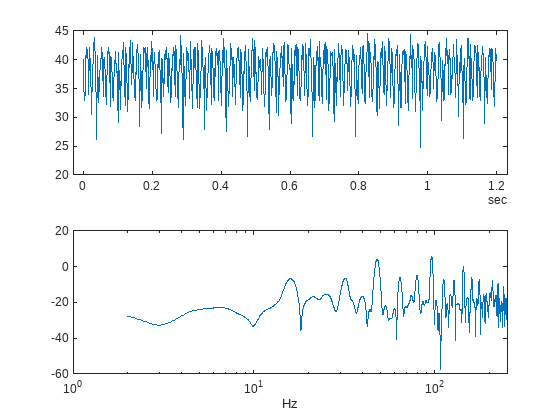The flow spectrum reveals resonant peaks at expected frequencies. Specifically, the pump motor speed is 950 rpm, or 15.833 Hz, and since the pump has 3 cylinders the flow is expected to have a fundamental at 3*15.833 Hz, or 47.5 Hz, as well as harmonics at multiples of 47.5 Hz. The flow spectrum clearly shows the expected resonant peaks. Faults in one cylinder of the pump will result in resonances at the pump motor speed, 15.833 Hz and its harmonics.

The flow spectrum and slow signal gives some ideas of possible condition indicators. Specifically, common signal statistics such as mean, variance, etc. as well as spectrum characteristics. Spectrum condition indicators relating to the expected harmonics such as the frequency with the peak magnitude, energy around 15.833 Hz, energy around 47.5 Hz, energy above 100 Hz, are computed. The frequency of the spectral kurtosis peak is also computed.

Configure the ensemble with data variables for the condition indicators and condition variables for fault variable values. Then call the `extractCI` function to compute the features, and use the `writeToLastMemberRead` command to add the feature and fault variable values to the ensemble. The `extractCI` function is defined at the end of this example.

```ens.DataVariables = [ens.DataVariables; ... "fPeak"; "pLow"; "pMid"; "pHigh"; "pKurtosis"; ... "qMean"; "qVar"; "qSkewness"; "qKurtosis"; ... "qPeak2Peak"; "qCrest"; "qRMS"; "qMAD"; "qCSRange"]; ens.ConditionVariables = ["LeakFault","BlockingFault","BearingFault"]; feat = extractCI(flow,flowP,flowF); dataToWrite = [faultValues, feat]; writeToLastMemberRead(ens,dataToWrite{:})```

The above code preprocesses and computes the condition indicators for the first member of the simulation ensemble. Repeat this for all the members in the ensemble using the ensemble `hasdata` command. To get an idea of the simulation results under different fault conditions plot every hundredth element of the ensemble.

```%Figure with nominal and faults figure, subplot(211); lFlow = plot(flow.Time,flow.Data,'LineWidth',2); subplot(212); lFlowP = semilogx(flowF,pow2db(flowP),'LineWidth',2); xlabel('Hz') ct = 1; lColors = get(lFlow.Parent,'ColorOrder'); lIdx = 2; % Loop over all members in the ensemble, preprocess % and compute the features for each member while hasdata(ens) % Read member data data = read(ens); % Preprocess and extract features from the member data [flow,flowP,flowF,faultValues] = preprocess(data); feat = extractCI(flow,flowP,flowF); % Add the extracted feature values to the member data dataToWrite = [faultValues, feat]; writeToLastMemberRead(ens,dataToWrite{:}) % Plot member signal and spectrum for every 100th member if mod(ct,100) == 0 line('Parent',lFlow.Parent,'XData',flow.Time,'YData',flow.Data, ... 'Color', lColors(lIdx,:)); line('Parent',lFlowP.Parent,'XData',flowF,'YData',pow2db(flowP), ... 'Color', lColors(lIdx,:)); if lIdx == size(lColors,1) lIdx = 1; else lIdx = lIdx+1; end end ct = ct + 1; end```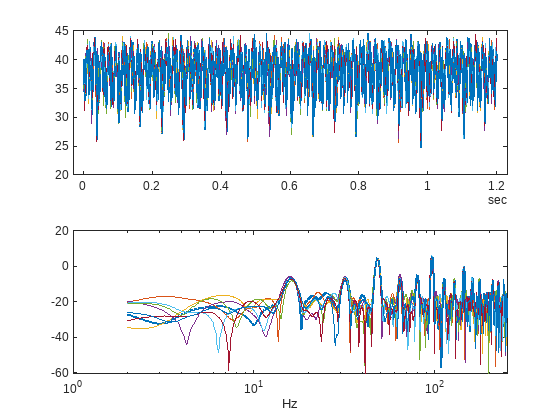Note that under different fault conditions and severities the spectrum contains harmonics at the expected frequencies.

### Detect and Classify Pump Faults

The previous section preprocessed and computed condition indicators from the flow signal for all the members of the simulation ensemble, which correspond to the simulation results for different fault combinations and severities. The condition indicators can be used to detect and classify pump faults from a pump flow signal.

Configure the simulation ensemble to read the condition indicators, and use the tall and gather commands to load all the condition indicators and fault variable values into memory

```% Get data to design a classifier. reset(ens) ens.SelectedVariables = [... "fPeak","pLow","pMid","pHigh","pKurtosis",... "qMean","qVar","qSkewness","qKurtosis",... "qPeak2Peak","qCrest","qRMS","qMAD","qCSRange",... "LeakFault","BlockingFault","BearingFault"]; idxLastFeature = 14; % Load the condition indicator data into memory data = gather(tall(ens));```
```Starting parallel pool (parpool) using the 'local' profile ... Preserving jobs with IDs: 1 2 because they contain crash dump files. You can use 'delete(myCluster.Jobs)' to remove all jobs created with profile local. To create 'myCluster' use 'myCluster = parcluster('local')'. connected to 6 workers. Evaluating tall expression using the Parallel Pool 'local': - Pass 1 of 1: Completed in 45 sec Evaluation completed in 45 sec ```
`data(1:10,:)`
```ans=10×17 table fPeak pLow pMid pHigh pKurtosis qMean qVar qSkewness qKurtosis qPeak2Peak qCrest qRMS qMAD qCSRange LeakFault BlockingFault BearingFault ______ _______ ______ ______ _________ ______ ______ _________ _________ __________ ______ ______ ______ ________ _________ _____________ ____________ 43.909 0.86472 117.63 18.874 276.49 35.572 7.5242 -0.72832 2.7738 13.835 1.1494 35.677 2.2326 42690 1e-09 0.8 0 43.909 0.44477 125.92 18.899 12.417 35.576 7.869 -0.7094 2.6338 13.335 1.1449 35.686 2.3204 42697 1e-09 0.8 0 43.909 1.1782 137.99 17.526 11.589 35.573 7.4367 -0.72208 2.7136 12.641 1.1395 35.678 2.2407 42695 1e-09 0.8 0 44.151 156.74 173.84 21.073 199.5 33.768 12.466 -0.30256 2.4782 17.446 1.2138 33.952 2.8582 40518 2.4e-06 0.8 0 43.848 0.71756 110.92 18.579 197.02 35.563 7.5781 -0.72377 2.793 14.14 1.1504 35.669 2.2671 42682 1e-09 0.8 0 43.909 0.43673 119.56 20.003 11.589 35.57 7.5028 -0.74797 2.7913 13.833 1.1551 35.676 2.2442 42689 1e-09 0.8 0 43.788 0.31617 135.3 19.724 476.82 35.568 7.4406 -0.70964 2.6884 14.685 1.1473 35.673 2.2392 42687 1e-09 0.8 0 43.848 0.72747 121.63 19.733 11.589 35.523 7.791 -0.72736 2.7864 14.043 1.1469 35.633 2.2722 42633 1e-09 0.8 0 43.848 0.62777 128.85 19.244 11.589 35.541 7.5698 -0.6953 2.6942 13.451 1.1415 35.647 2.2603 42654 1e-09 0.8 0 43.848 0.4631 134.83 18.918 12.417 35.561 7.8607 -0.68417 2.6664 13.885 1.1504 35.671 2.3078 42681 1e-09 0.8 0 ```

The fault variable values for each ensemble member (row in the data table) can be converted to fault flags and the fault flags combined to single flag that captures the different fault status of each member.

```% Convert the fault variable values to flags data.LeakFlag = data.LeakFault > 1e-6; data.BlockingFlag = data.BlockingFault < 0.8; data.BearingFlag = data.BearingFault > 0; data.CombinedFlag = data.LeakFlag+2*data.BlockingFlag+4*data.BearingFlag;```

Create a classifier that takes as input the condition indicators and returns the combined fault flag. Train a support vector machine that uses a 2nd order polynomial kernel. Use the `cvpartition` command to partition the ensemble members into a set for training and a set for validation.

```rng('default') % for reproducibility predictors = data(:,1:idxLastFeature); response = data.CombinedFlag; cvp = cvpartition(size(predictors,1),'KFold',5); % Create and train the classifier template = templateSVM(... 'KernelFunction', 'polynomial', ... 'PolynomialOrder', 2, ... 'KernelScale', 'auto', ... 'BoxConstraint', 1, ... 'Standardize', true); combinedClassifier = fitcecoc(... predictors(cvp.training(1),:), ... response(cvp.training(1),:), ... 'Learners', template, ... 'Coding', 'onevsone', ... 'ClassNames', [0; 1; 2; 3; 4; 5; 6; 7]);```

Check the performance of the trained classifier using the validation data and plot the results on a confusion plot.

```% Check performance by computing and plotting the confusion matrix actualValue = response(cvp.test(1),:); predictedValue = predict(combinedClassifier, predictors(cvp.test(1),:)); confdata = confusionmat(actualValue,predictedValue); figure, labels = {'None', 'Leak','Blocking', 'Leak & Blocking', 'Bearing', ... 'Bearing & Leak', 'Bearing & Blocking', 'All'}; h = heatmap(confdata, ... 'YLabel', 'Actual leak fault', ... 'YDisplayLabels', labels, ... 'XLabel', 'Predicted fault', ... 'XDisplayLabels', labels, ... 'ColorbarVisible','off');```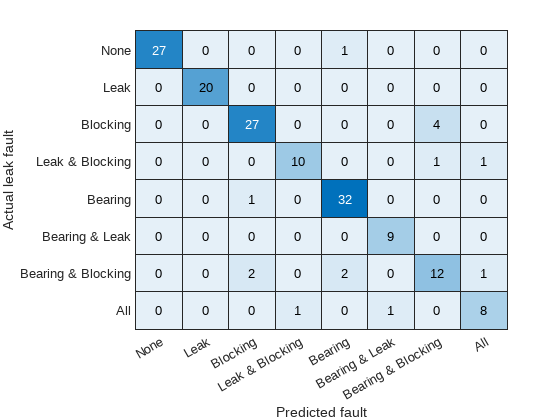The confusion plot shows for each combination of faults the number of times the fault combination was correctly predicted (the diagonal entries of the plot) and the number of times the fault combination was incorrectly predicted (the off-diagonal entries).

The confusion plot shows that the classifier did not correctly classify some fault conditions (the off diagonal terms). However, the no fault condition was correctly predicted. In a couple of places a no fault condition was predicted when there was a fault (the first column), otherwise a fault was predicted although it may not be exactly the correct fault condition. Overall the validation accuracy was 84% and the accuracy at predicting that there is a fault 98%.

```% Compute the overall accuracy of the classifier sum(diag(confdata))/sum(confdata(:))```
```ans = 0.6150 ```
```% Compute the accuracy of the classifier at predicting % that there is a fault 1-sum(confdata(2:end,1))/sum(confdata(:))```
```ans = 0.9450 ```

Examine the cases where no fault was predicted but a fault did exist. First find cases in the validation data where the actual fault was a blocking fault but a no fault was predicted.

```vData = data(cvp.test(1),:); b1 = (actualValue==2) & (predictedValue==0); fData = vData(b1,15:17)```
```fData=11×3 table LeakFault BlockingFault BearingFault _________ _____________ ____________ 1e-09 0.77 0 1e-09 0.77 0 1e-09 0.71 0 1e-09 0.77 0 1e-09 0.77 0 1e-09 0.62 0 1e-09 0.77 0 1e-09 0.77 0 1e-09 0.71 0 8e-07 0.74 0 1e-09 0.74 0 ```

Find cases in the validation data where the actual fault was a bearing fault but a no fault was predicted.

```b2 = (actualValue==4) & (predictedValue==0); vData(b2,15:17)```
```ans = 0×3 empty table ```

Examining the cases where no fault was predictive but a fault did exist reveals that they occur when the blocking fault value of 0.77 is close to its nominal value of 0.8, or the bearing fault value of 6.6e-5 is close to its nominal value of 0. Plotting the spectrum for the case with a small blocking fault value and comparing with a fault free condition reveals that spectra are very similar making detection difficult. Re-training the classifier but including a blocking value of 0.77 as a non fault condition would significantly improve the performance of the fault detector. Alternatively, using additional pump measurements could provide more information and improve the ability to detect small blocking faults.

```% Configure the ensemble to only read the flow and fault variable values ens.SelectedVariables = ["qOut_meas","LeakFault","BlockingFault","BearingFault"]; reset(ens) % Load the ensemble member data into memory data = gather(tall(ens));```
```Evaluating tall expression using the Parallel Pool 'local': - Pass 1 of 1: Completed in 38 sec Evaluation completed in 38 sec ```
```% Look for the member that was incorrectly predicted, and % compute its power spectrum idx = ... data.BlockingFault == fData.BlockingFault(1) & ... data.LeakFault == fData.LeakFault(1) & ... data.BearingFault == fData.BearingFault(1); flow1 = data(idx,1); flow1 = flow1.qOut_meas{1}; [flow1P,flow1F] = pspectrum(flow1); % Look for a member that does not have any faults idx = ... data.BlockingFault == 0.8 & ... data.LeakFault == 1e-9 & ... data.BearingFault == 0; flow2 = data(idx,1); flow2 = flow2.qOut_meas{1}; [flow2P,flow2F] = pspectrum(flow2); % Plot the power spectra semilogx(... flow1F,pow2db(flow1P),... flow2F,pow2db(flow2P)); xlabel('Hz') legend('Small blocking fault','No fault')```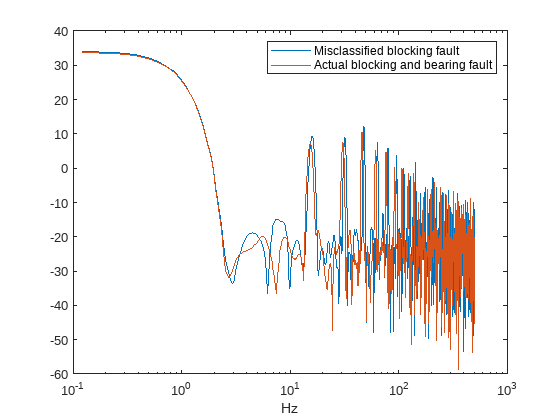### Conclusion

This example showed how to use a Simulink model to model faults in a reciprocating pump, simulate the model under different fault combinations and severities, extract condition indicators from the pump output flow and use the condition indicators to train a classifier to detect pump faults. The example examined the performance of fault detection using the classifier and noted that small blocking faults are very similar to the no fault condition and cannot be reliably detected.

### Supporting Functions

```function [flow,flowSpectrum,flowFrequencies,faultValues] = preprocess(data) % Helper function to preprocess the logged reciprocating pump data. % Remove the 1st 0.8 seconds of the flow signal tMin = seconds(0.8); flow = data.qOut_meas{1}; flow = flow(flow.Time >= tMin,:); flow.Time = flow.Time - flow.Time(1); % Ensure the flow is sampled at a uniform sample rate flow = retime(flow,'regular','linear','TimeStep',seconds(1e-3)); % Remove the mean from the flow and compute the flow spectrum fA = flow; fA.Data = fA.Data - mean(fA.Data); [flowSpectrum,flowFrequencies] = pspectrum(fA,'FrequencyLimits',[2 250]); % Find the values of the fault variables from the SimulationInput simin = data.SimulationInput{1}; vars = {simin.Variables.Name}; idx = strcmp(vars,'leak_cyl_area_WKSP'); LeakFault = simin.Variables(idx).Value; idx = strcmp(vars,'block_in_factor_WKSP'); BlockingFault = simin.Variables(idx).Value; idx = strcmp(vars,'bearing_fault_frict_WKSP'); BearingFault = simin.Variables(idx).Value; % Collect the fault values in a cell array faultValues = {... 'LeakFault', LeakFault, ... 'BlockingFault', BlockingFault, ... 'BearingFault', BearingFault}; end function ci = extractCI(flow,flowP,flowF) % Helper function to extract condition indicators from the flow signal % and spectrum. % Find the frequency of the peak magnitude in the power spectrum. pMax = max(flowP); fPeak = flowF(flowP==pMax); % Compute the power in the low frequency range 10-20 Hz. fRange = flowF >= 10 & flowF <= 20; pLow = sum(flowP(fRange)); % Compute the power in the mid frequency range 40-60 Hz. fRange = flowF >= 40 & flowF <= 60; pMid = sum(flowP(fRange)); % Compute the power in the high frequency range >100 Hz. fRange = flowF >= 100; pHigh = sum(flowP(fRange)); % Find the frequency of the spectral kurtosis peak [pKur,fKur] = pkurtosis(flow); pKur = fKur(pKur == max(pKur)); % Compute the flow cumulative sum range. csFlow = cumsum(flow.Data); csFlowRange = max(csFlow)-min(csFlow); % Collect the feature and feature values in a cell array. % Add flow statistic (mean, variance, etc.) and common signal % characteristics (rms, peak2peak, etc.) to the cell array. ci = {... 'qMean', mean(flow.Data), ... 'qVar', var(flow.Data), ... 'qSkewness', skewness(flow.Data), ... 'qKurtosis', kurtosis(flow.Data), ... 'qPeak2Peak', peak2peak(flow.Data), ... 'qCrest', peak2rms(flow.Data), ... 'qRMS', rms(flow.Data), ... 'qMAD', mad(flow.Data), ... 'qCSRange',csFlowRange, ... 'fPeak', fPeak, ... 'pLow', pLow, ... 'pMid', pMid, ... 'pHigh', pHigh, ... 'pKurtosis', pKur(1)}; end ```